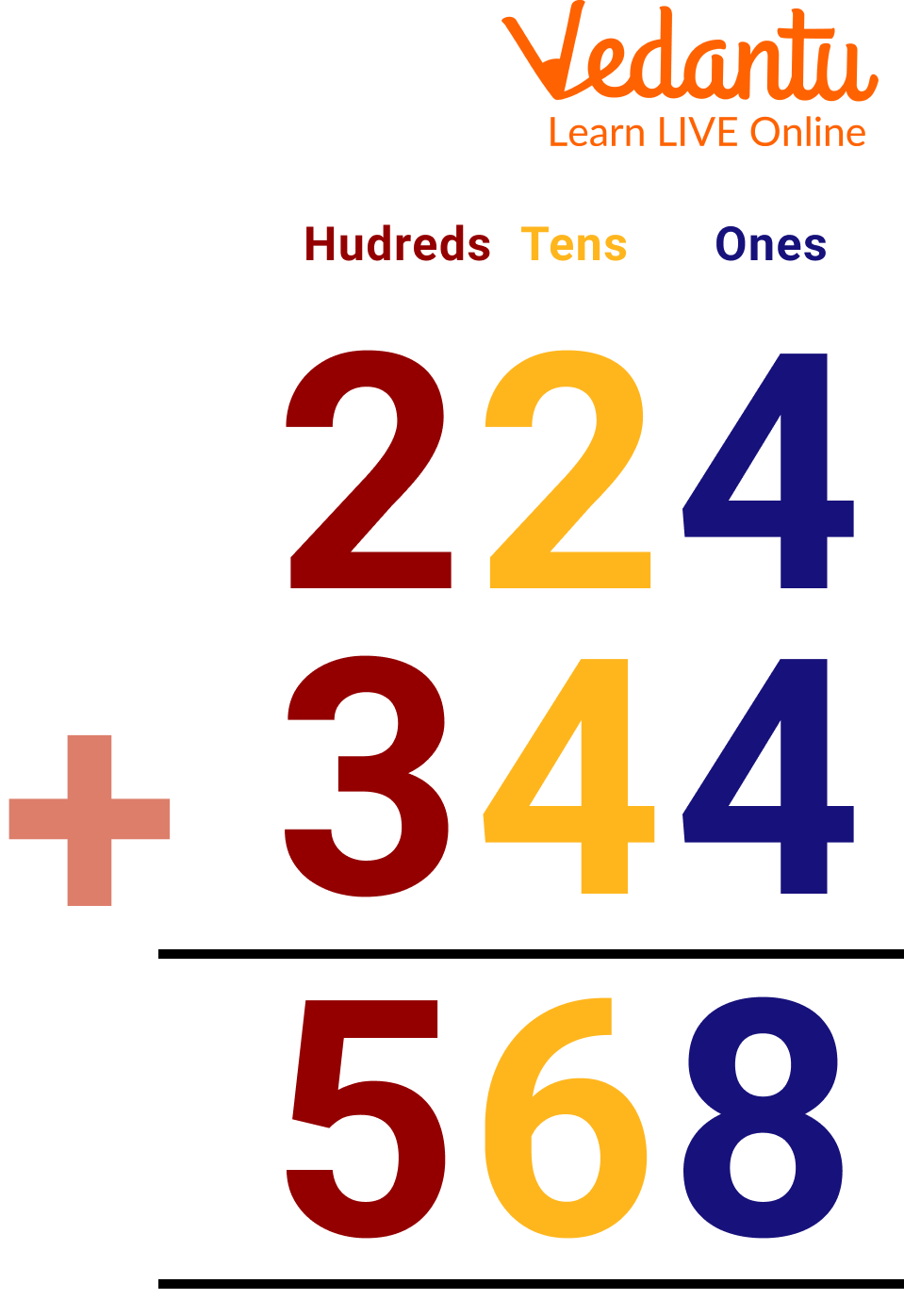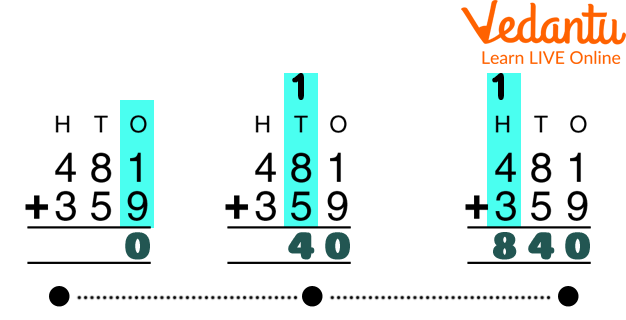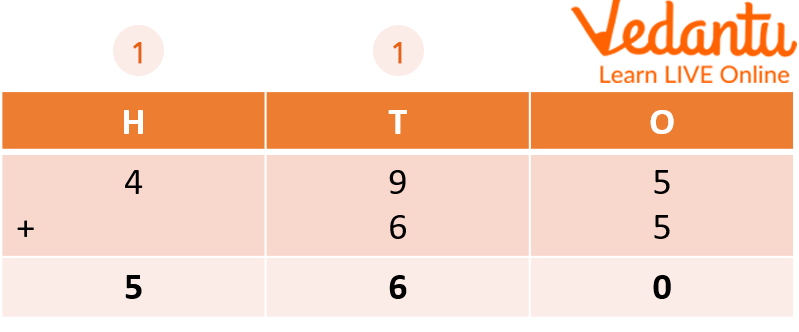Courses
Courses for Kids
Free study material
Free LIVE classes
MorePutting things together is done by addition. Regrouping is known as arranging numbers in columns of tens and ones for addition. Addition with regrouping is also known as carrying addition. In addition, with regrouping, we arrange two or more large numbers column by column according to their place value, and when the sum of any of these columns exceeds 9, we regroup that sum into tens and ones. In this article, we will learn 3-digit addition with regrouping plans where we will learn about 3-digit addition and its regrouping.3-Digit Addition with Regrouping Word Problem

In 3-digit addition, we need to place the given numbers into columns according to their respective place values, like ones, tens, hundreds, thousands, and so on. It should be remembered that the numbers being added are known as addends, and the result of adding these numbers is known as their sum.If you are adding three-digit integers, you should always add your digits in the one's place first, then the tens place, and finally the hundreds place. Reassessing your situation enables you to determine whether you have too much, just enough, or not enough of something. Remember that in case of 10 or more, put it next door whenever you need to regroup. So, for solving, first, arrange numbers according to their place value, then add one place, then tens place, then hundreds place, and so on.3-digit Addition with Regrouping Word Problems

## Steps for 3-Digit Addition with Regrouping

Following are the steps for the addition of 3-digit numbers with regrouping:

Let us add 295 +143 using the following steps:

Step 1: Arrange 295 and 143 into columns based on their place values. As a result, the numbers 5 and 3 will appear in the ones column, 9 and 4 in the tens column, and 2 and 1 in the hundreds column.

Step 2: Beginning with the ones column, we may add the digits to get 5 + 3 = 8.

Step 3: Now let's add the numbers in the tens column: 9 + 4 = 13. We need to regroup it because 13 is greater than 9. As a result, we enter 3 in this column and move 1 to the following column.

Step 4: The numbers and the carried-over number are now added in the hundreds column. It will therefore be 2 + 1 + 1 = 4. Therefore, we enter 4 as the total for this column. Consequently, 295 + 143 = 438.

## How to Teach 3-Digit Addition with Regrouping?

Regrouping, also known as carrying and borrowing, is forming groups of tens when adding or subtracting two-digit numbers (or more). When initially introducing it, it is important to utilise physical manipulatives and connect regrouping to place value.

## Solved Examples

1. Solve 495 + 65.

Ans:Solved Example

Ans: we get 295 + 143 = 438

Here, 5 and 3 at one place, 9 and 4 at the tens place, and 2 and 1 at the hundreds place. Now, add 5 + 3 = 8, then tens column, i.e., 9 + 4 = 13 then carry 1 to the next column, as these are two numbers, then add 2 + 1 + 1 = 4. So, we get 438.

Ans: We get 155 + 123 = 278.

Here, 5 and 3 at one place, 5 and 2 at the tens place, and 1 and 1 at the hundreds place. Now, add 5 + 3 = 8 then tens column, i.e., 5 + 2 = 7, and then add 1 + 1 = 1. We get 278.

Ans: Adding ones place, we get 8 + 3 = 11, so carry 1 at tens place, then add tens place we get 6 + 2 + 1 = 9. Thus, we have 68 + 23 = 91.

5. Add the smallest and largest three-digit number.

Ans: The smallest three-digit number is 100.

The largest three-digit number is 999.

By adding them, we get 1099.

## Practice Questions

Ans: 232

Ans: 1050

Ans: 612

4. State true or false: 650 + 420 = 1070.

Ans: True

5. By using regrouping, add 549 and 496.

Ans: 1045

## Summary

The addition of 3-digit numbers with regrouping in Mathematics refers to converting groupings of ones into tens to facilitate addition and subtraction. Additionally, if the numbers you are adding result in a two-digit number and are not in the farthest left column, you must regroup. When adding integers, be sure that at least one addend contains three digits. By placing the three-digit integers in columns according to their place values, we can add them. Then, after combining the numbers from the ones and tens columns, we go to the hundreds column.

I hope after reading this article, you will now have full command over the topic. If you still find any issues, do check out our other articles. You can also download 3-digit addition with a regrouping pdf.

Last updated date: 16th Sep 2023
Total views: 105.9k
Views today: 3.05k

## FAQs on 3-Digit Addition with Regrouping

1. How are 3-digit addition word problems different from sums?

3-digit addition word problems have a language and theory. In those problems, one has to solve the sum situationally. Kids don’t have to solve the sums directly, they first understand the language and then solve them. This has extra benefits to kids.

2. Why is 3-digit addition with regrouping important to learn?

3-digit addition with regrouping is important for kids as it helps them to learn the systematic way of adding the values, it also helps the kids in mastering the place values.

3. How can the kids master the addition of 3-digit numbers with regrouping?

The addition of 3-digit numbers with regrouping can be mastered by solving a 3-digit addition pdf consisting of sums. Worksheets can also be solved to practise addition sums.